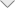### Fractal Antenna Simulation

Introduction

This application demonstrates modeling of fractal antennas within EMC Studio/EMCoS Antenna VLab environment. The triangular shaped fractal, Sierpinski gasket monopole antenna is considered. Structure of this antenna is intended to be used for four discrete frequency bands.
Simulated resonance frequencies of the Sierpinski gasket monopole antenna are compared with analytical solution.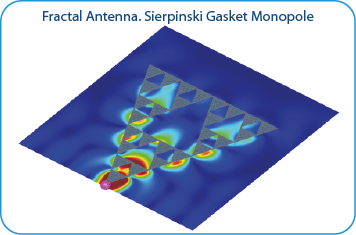Simulation Model Description

Sierpinski gasket with largest side length of 102.7683 mm or a height of 88.9 mm is considered. The substrate thickness is 1.588 mm, and relative permittivity is 2.5.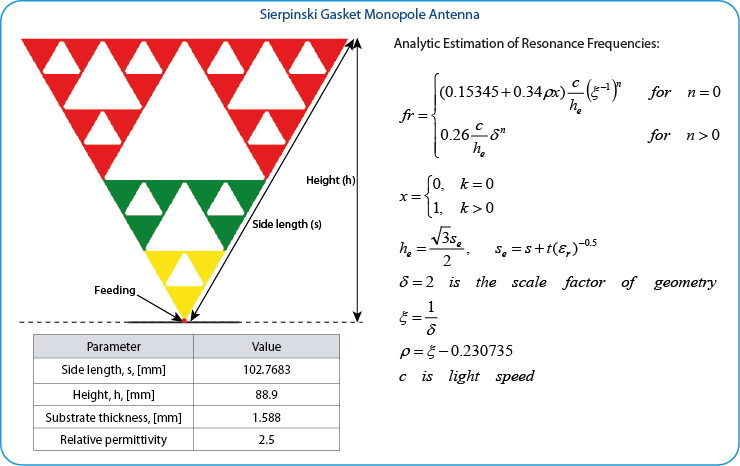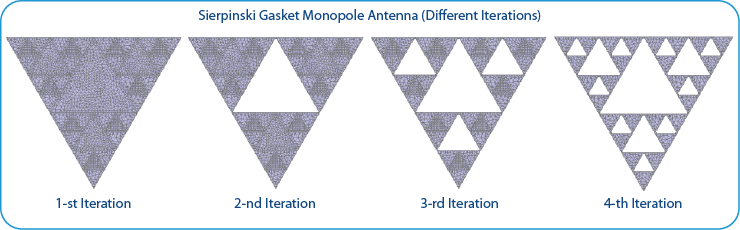Results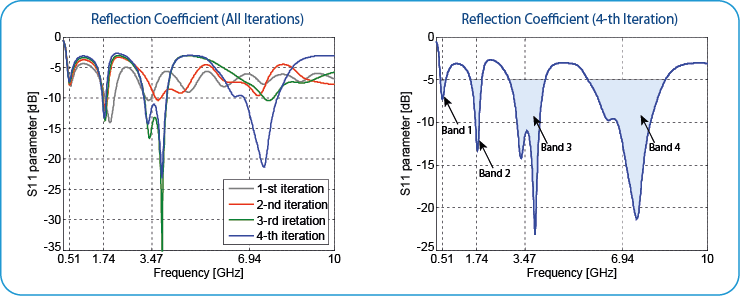The resonance frequencies of the Sierpinski gasket monopole antenna obtained from the analytical formulas and simulated in EMC Studio/EMCoS Antenna VLab are shown in table.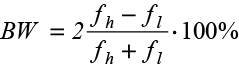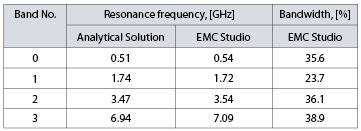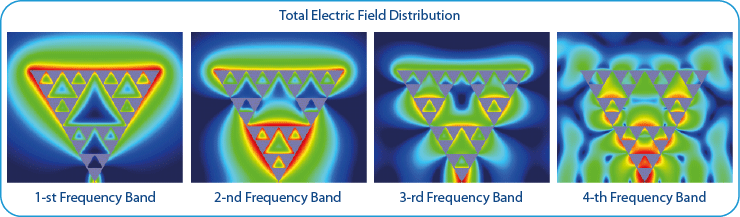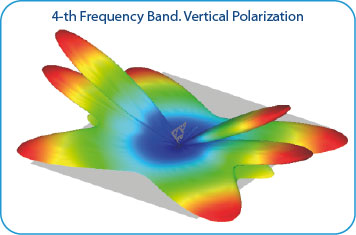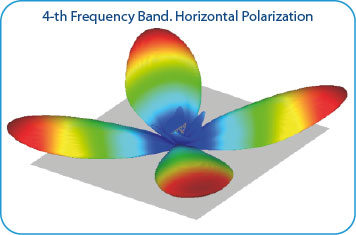Conclusions

• Fractal antenna simulations can be effectively done in EMC Studio and EMCoS Antenna VLab environmen
• Simulated characteristics of Sierpinski gasket monopole antenna are in good agreement with analytical results

References

• R. K. Mishra, R. Ghatak, and D. R. Poddar, “Design formula for Sierpinski gasket pre-fractal planar-monopole antennas”, IEEE Antennas Propag. Mag., vol. 50, no. 3, pp. 104-107, Jun. 2008
﻿